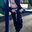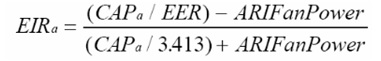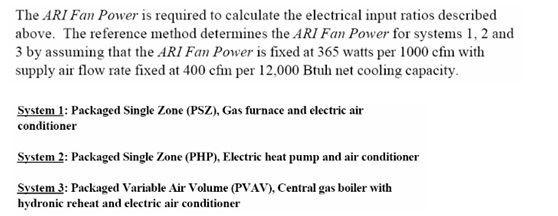Question-and-Answer Resource for the Building Energy Modeling Community
Get s tarted with the Help page

# Convert SEER to EER for eQUESTI am sure this question has been asked many times before in the onebuilding list but I wanted to have it answered here as well. When ASHRAE 90.1 or MNECB/CNEB ask for a performance rating for a cooling system in SEER, how does one convert this SEER value into an EER to be used in eQUEST?

I know equest uses some formula of conversion in the wizards however where does this formula come from? is it really applicable? I am interested in hearing some thoughts on this subject.

Thanks!

Jason

edit retag close merge delete

Sort by » oldest newest most voted

Just noticed a 2013 paper from Texas A&M, METHODOLOGY FOR CALCULATING COOLING AND HEATING ENERGY-INPUT-RATIO (EIR) FROM THE RATED SEASONAL PERFORMANCE EFFICIENCY (SEER OR HSPF). That paper presents correlations to give you COP as a function of SEER. They report this correlation works better than earlier methods from FSEC and ASHRAE RP-1197.

Find it here: http://repository.tamu.edu/handle/196...

The paper is marked DRAFT. Perhaps one of the authors can report on status.

-Erik

more

2

These formulae are published in ASHRAE 90.1-2013, G3.1.2.1.Two formulas that I've used in the past:

EER approximately equal to 0.9 * SEER
EER = -0.02SEER^2 + 1.12SEER

The first is just from general googling, and was a rough consensus value between several sources. The second is from the eQuest mailing list forums. Using a SEER of 10 gives you 9 from the first, and 9.2 from the second.

more

I believe the second equation can be found in TRANE hvac equipment literature. It is the one we typically use.

EIR is used in eQUEST,all the EER/SEER/HSPF will be converted to EIR finally.where
CAP Total Cooling Capacity(Btu/h)
EER ARI Rated Energy Efficenice RatioCooling EIR=(1/EER-0.012167)/((1/3.413)+0.012167)
Where
the constant 0.012167=(365/1000)(400/12000)
EER=SEER
0.778+1.4

Heating EIR = (1/(COP3.413)-0.012167)/((1/3.413)-0.012167)
Heating EIR = (1/((HSPF
0.28+1.13)*3.413)-0.012167)/((1/3.413)-0.012167)

more